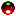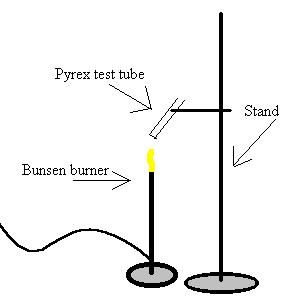CH - 120

Fall 2000

Stoichiometry

Experiment #4

Submitted by:

Andrew J. Buettner

Partner:

none

Report Due: March 6, 2003Objective

The Objective of this lab is to use stoichiometry to determine the amount of a substance in a mixture. In this case it is potassium chlorate in potassium chloride.Apparatus

A) Equipment used

1) Pyrex test tube

2) Manganese Dioxide

3) Potassium Chlorate

4) Stand

5) Bunsen Burner

6) Unknown mixture #1

B) Diagram:Method

A: Part 1

1) Add a small amount of manganese dioxide to a Pyrex test tube and mass the total

2) Add about 1g of Potassium Chlorate to the test tube and mass again

3) Heat the Pyrex test tube and it's contents until the solid melts and dries again

4) Mass the Pyrex test tube after it has cooled

B: Part 2

1) Clean the Pyrex test tube and add another small amount of manganese to the test tube.

2) Mass the test tube and it's contents

3) Add about 1g of unknown mixture #1 to the test tube and mass again

4) Heat the test tube until the solid melts and re-solidifies

5) Mass the contentsData

A: Part 1 Results:

1) Tube with manganese: 31.23g

2) Tube with everything: 3273g

3) Mass after burn: 32.37g

B: Part 2 Results:

4) Test tube with manganese: 31.90g

5) Test tube with mixture: 32.93g

6) Mass after burn: 32.65gResults

A: Part 1

1) Mass of oxygen lost: .36g

2) Moles of oxygen lost: .011mole

3) Mass of potassium chloride remaining: 1.14g

4) Moles of potassium chloride: .0153mole

5) Moles of potassium initially: .0153mole

6) Moles of chlorine initially: .0153mole

7) Moles of oxygen initially .011mole

8) Simplest formula of potassium chlorate: K2Cl2O3

B: Part 2:

9) Mass of unknown: 1.03g

10) Mass of oxygen lost: .28g

11) Moles of oxygen lost: .00875mole

12) Moles of potassium chlorate decomposed: .0113mole

13) Mass of potassium chlorate initially: .859g

14) Potassium chlorate percent weight: 81.5%Answers to Lab Questions

1) Q: How would a small amount of water present in the sample of potassium chlorate affect the calculated ratio of oxygen to chlorine atoms?

A: The ratio would decrease as the mass lost to oxygen would increase

2) Q: What would be the maximum mass of oxygen released if .950g KclO4 were reacted?

A: .439gO2

3) Q: KCl can sublime when heated, how would this affect the results in part A?

A: It would decrease the calculated mass of O2 given off therefore widening the ratio between O2 and Cl.

4) Q: If a 1.10g mixture containing 75% potassium chlorate and 25% non reactive materials, what would be the expected loss in mass due to oxygen?

A: .261g lostConclusions

From this lab, using stoichiometry, it can be determined that there was 81.5% potassium chlorate by weight mixed in with potassium chloride. The percent error of this calculation is unable to be determined.Attachments

1) Lab data

2) Calculations